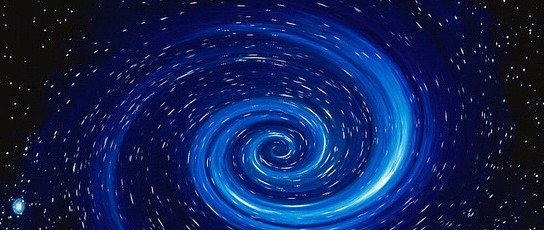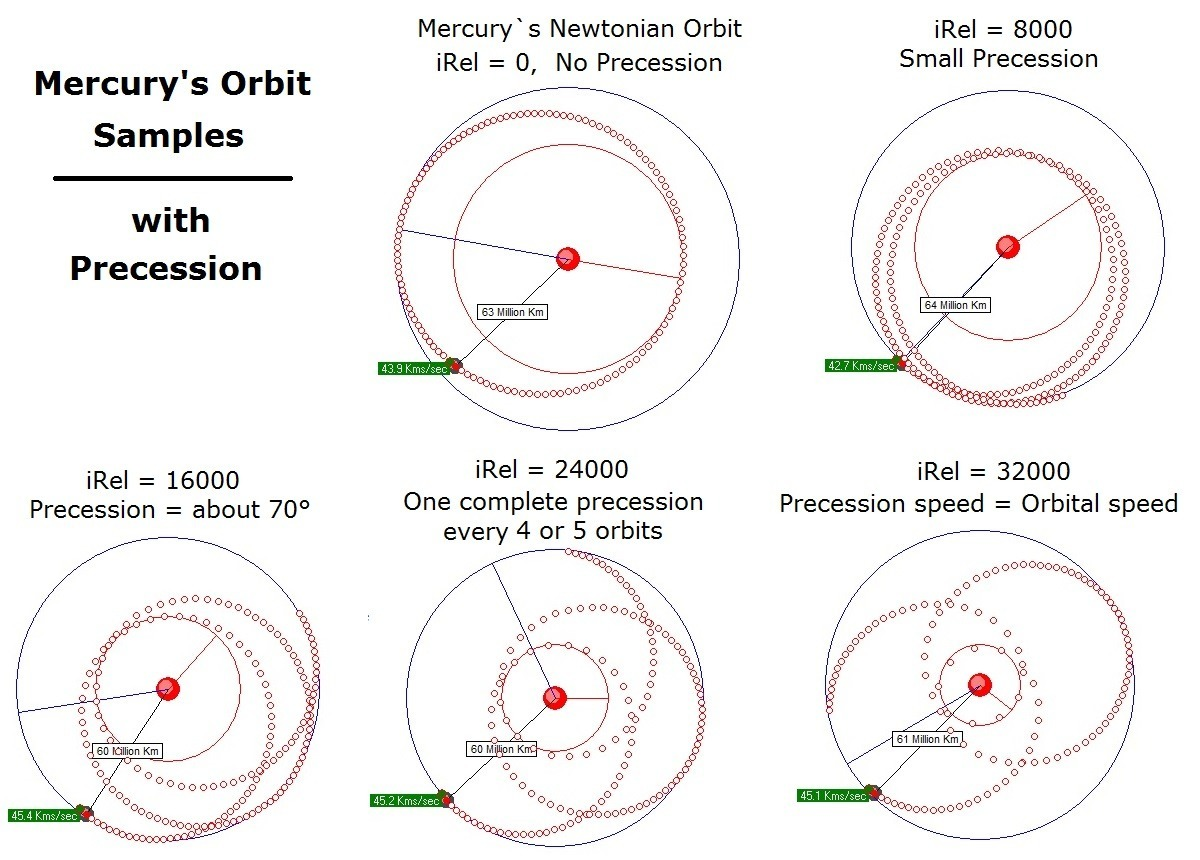# Adding Relativity to Gravity AnimationsMy last entry dealt with lessons learned while building a gravity animation. The animation works just like frames in a movie. Between each frame, the gravitational force between objects is calculated displayed on the screen as “Force”. This force is added to the current motion (momentum) of the object. The motion is added to the prior location of the object to get the new location of the object. The force on each object was found using the gravitational inverse square law F=G*(M₁*M₂) / D².

This works pretty well and you can see a simulated orbit of Mercury on this link. Running the animation produces a pretty good model of Mercury’s orbit, ranging from 69.4 to 46.4 million km, with a year being a fraction over 88 days (Mercury actual aphelion is 69.8, perihelion is 46.o million km, period is just under 88 days). The starting position, momentum and mass of Mercury and the sun were all done with 3 digits of accuracy and the calculation frame rate was just under every 9 hours. The results are good and you can visualize that with a faster calculation rate and more accurate starting conditions, you could get as much accuracy as you would want.

But, unfortunately no matter how accurate we make it, it will never work. Einstein’s work with relativity, tells us that there is more to gravity then meets the eye. In fact, observations tell us that Mercury’s orbit cannot be properly modeled with the gravitational inverse square law no matter the accuracy. How do we model Mercury’s orbit properly? The Newtonian calculations used are pretty straight forward, but under relativity, things become more complex. An article at Mathpages.com on Relativity shows the calculations used to get from Einstein’s field equations to the amount of precession in an orbit.It is the task of the animator to produce results in the shortest amount of time with the least amount of work. It turns out that it is incredibly easy to produce an animation with precession. Under Newton, we calculate the force on M₁ as G*M₂/D². To produce a precession, we add a cubed factor, where the force on M₁ is G*M₂/D² + iRel*M₂/D³. The number iRel is just a number we make up to indicate how fast the precession should move. Wow, that’s pretty easy and we can get a precession of Mercury at whatever speed we want with whatever accuracy we want.

But, not so fast. To put the precession rates into perspective, suppose the sun was compressed into a tiny, tiny space from it’s current radius of 696,000 kms. This would make the sun a black hole with a 3 km event horizon. We are talking about orbiting objects around the mass of the sun, at a distance approaching 4.5 kms. If any orbit gets within 1.5 times the event horizon (called the light orbit), it will be sucked into the center of the black hole, into a singularity.

At this point, the animation breaks down completely. The force between the singularity and another object becomes stronger and stronger as the object approaches closer and closer. Suddenly the object jumps to some random spot way over on the other side of the singularity. You can improve things by moving to a faster frame rate, but that is only a temporary solution. There is no limit to the force between the singularity and the object, so there  is no limit to how fast you have to make the frame rate to model this type of behavior.

The problem is not limited to relativity.  Using the gravitational inverse square law where we point two objects momentum directly at each other, we have the same problem where there is no limit on the force between the objects. Coulomb’s law that governs the attraction between protons and electrons has the same problem. A stationary proton and electron will slowly attract each other, but as the electron gains speed and gets closer to the proton, there is no limit to the force that the proton will exert on the electron. Faster and faster frame rates with ever more accurate calculations do not resolve the problem as there is no limit to the force the electron feels as it gets closer and closer to the proton.

What can we do? Can we properly model gravity singularities using an animated model? Can we model proton/electron interactions? Sure we can. Relativity is not just about precession. There is an entire field of quantum mechanics that is meant to model interactions on an atomic scale. A closer look at both is pretty revealing.

Coming soon – A look at the fundamental differences between Newton’s gravity, Einstein’s gravity and quantum mechanics.